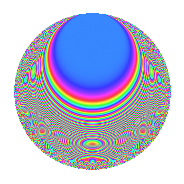# Properties

 Label 175.2.nLevel 175 Weight 2 Character orbit n Rep. character $$\chi_{175}(29,\cdot)$$ Character field $$\Q(\zeta_{10})$$ Dimension 56 Newform subspaces 1 Sturm bound 40 Trace bound 0

# Related objects

## Defining parameters

 Level: $$N$$ = $$175 = 5^{2} \cdot 7$$ Weight: $$k$$ = $$2$$ Character orbit: $$[\chi]$$ = 175.n (of order $$10$$ and degree $$4$$) Character conductor: $$\operatorname{cond}(\chi)$$ = $$25$$ Character field: $$\Q(\zeta_{10})$$ Newform subspaces: $$1$$ Sturm bound: $$40$$ Trace bound: $$0$$

## Dimensions

The following table gives the dimensions of various subspaces of $$M_{2}(175, [\chi])$$.

Total New Old
Modular forms 88 56 32
Cusp forms 72 56 16
Eisenstein series 16 0 16

## Trace form

 $$56q + 12q^{4} - 6q^{5} + 6q^{9} + O(q^{10})$$ $$56q + 12q^{4} - 6q^{5} + 6q^{9} - 4q^{10} + 8q^{11} - 40q^{12} - 4q^{14} - 18q^{15} - 32q^{16} + 12q^{19} + 12q^{20} + 4q^{21} - 30q^{22} + 10q^{23} - 28q^{24} + 4q^{25} + 12q^{26} + 30q^{27} - 2q^{29} + 28q^{30} + 12q^{31} + 20q^{33} + 2q^{35} - 14q^{36} - 70q^{37} - 70q^{38} - 4q^{39} - 30q^{40} + 4q^{41} + 50q^{42} + 22q^{44} - 52q^{45} - 4q^{46} - 10q^{47} + 30q^{48} - 56q^{49} - 54q^{50} - 44q^{51} - 20q^{53} + 54q^{54} - 2q^{55} + 12q^{56} + 10q^{58} - 6q^{59} + 16q^{60} - 4q^{61} + 50q^{62} + 20q^{63} + 24q^{64} - 18q^{65} - 74q^{66} + 10q^{67} - 78q^{69} + 8q^{70} - 8q^{71} + 140q^{72} + 40q^{73} + 60q^{74} - 8q^{75} + 52q^{76} - 20q^{77} - 90q^{78} + 124q^{80} - 72q^{81} - 30q^{83} - 12q^{84} + 96q^{85} - 20q^{86} + 30q^{87} + 140q^{88} + 38q^{89} - 8q^{90} + 8q^{91} + 80q^{92} + 88q^{94} - 70q^{95} - 28q^{96} - 30q^{97} + 60q^{99} + O(q^{100})$$

## Decomposition of $$S_{2}^{\mathrm{new}}(175, [\chi])$$ into newform subspaces

Label Dim. $$A$$ Field CM Traces $q$-expansion
$$a_2$$ $$a_3$$ $$a_5$$ $$a_7$$
175.2.n.a $$56$$ $$1.397$$ None $$0$$ $$0$$ $$-6$$ $$0$$

## Decomposition of $$S_{2}^{\mathrm{old}}(175, [\chi])$$ into lower level spaces

$$S_{2}^{\mathrm{old}}(175, [\chi]) \cong$$ $$S_{2}^{\mathrm{new}}(25, [\chi])$$$$^{\oplus 2}$$

## Hecke Characteristic Polynomials

There are no characteristic polynomials of Hecke operators in the database